# OECD Threatened species data analysis 5 - Bootstrap for Cinfidence Interval

This blog is following of

In this blog, I will show you how to get confidence interval with bootstrap method.

for BIRD, 95% confidence interval is 18.1 ~ 25.3 by parametric calculation.

average ± qt(0.975, d.f.)*S.E.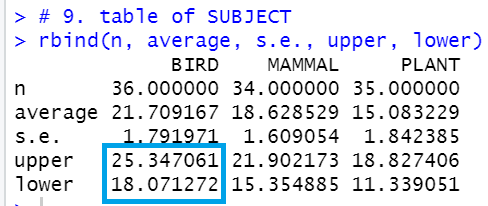We will calculate it with bootstrap method.

1. make a vector for BIRD.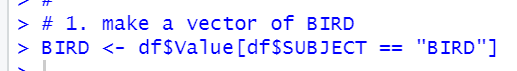2. decide how many times calculate average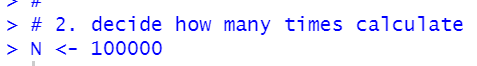I set it 100,000 times.

3. make a vector to stroe averages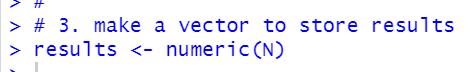4. make a function to draw random samples and calculate averages.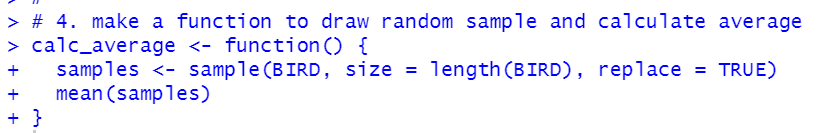let's check if the function works well.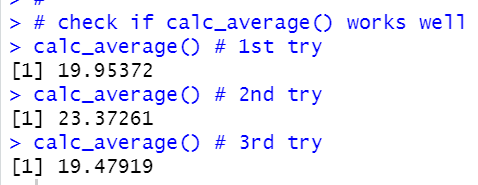we see 1st, 2nd and 3rd are different value, it means the function works fine.

5. use for() function to makes averages.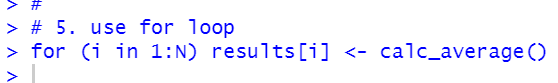6. use quantile() to get confidence interval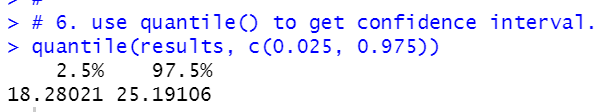That's it.

Let's make a histogram for results and vertical lines for confidence intervals.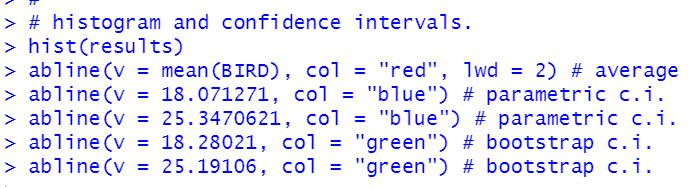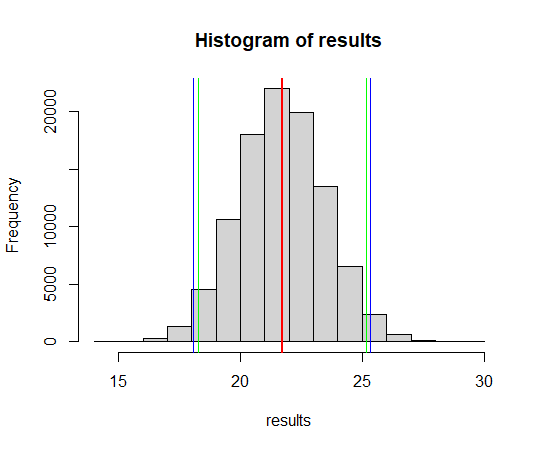The red vertical line is average,

the blue vertical lines are confidence interval by parametric and

the green vertical lines are confidence interval by bootstrap.

Thank you.